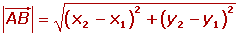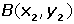Learn from home

The teachersA vector is a line segment running from point A (tail) to point B (head).Each vector has a magnitude (also referred to as length) and a direction.

### Direction of a Vector

This is the direction of the line which contains the vector or any line which is parallel to it.

### Magnitude of a VectorThe magnitude of the vectoris the length of the line segment. It is denoted by.

The magnitude of a vector is always a positive number or zero.The magnitude of a vector can be calculated if the coordinates of the endpoints are known:Calculate the magnitude of the following vectors:Calculate the value of k knowing the magnitude of the vector= (k, 3) is 5.### Position VectorThe vectorthat joins the coordinates origin, O, with a point, P, is the position vector of the point P.

### Components or Coordinates of a VectorIf the coordinates of A and B are:Find the components of the vector:The vectorhas the components (5, −2). Find the coordinates of A if the terminal point is known as B(12, −3).Calculate the coordinates of Point D so that the quadrilateral of Points: A(−1, −2), B(4, −1), C(5, 2) and D form a parallelogram.Did you like the article?(1 votes, average: 5.00 out of 5)Loading...

Emma

I am passionate about travelling and currently live and work in Paris. I like to spend my time reading, gardening, running, learning languages and exploring new places.

Did you like
this resource?

Bravo!